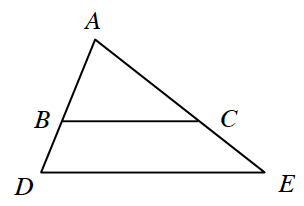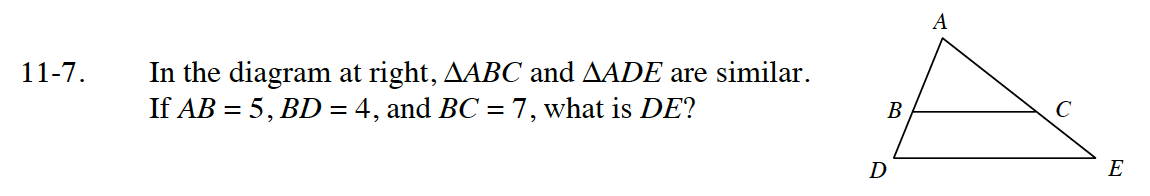Home > CC4 > Chapter 11 > Lesson 11.1.1 > Problem11-7

11-7.
1.In the diagram at right, ΔABC and ΔADE are similar. If AB = 5, BD = 4, and BC = 7, what is DE? Homework Help ✎Separate the triangles into two similar triangles.

What is the length of AD? Once you know this, since the triangles are similar, the ratios of corresponding sides are equal.
Remember to set up proportions.

DE = 12.6